# Bipolar Junction Transistor (BJT) theory

## Discrete Semiconductor Devices and Circuits

• #### Question 1

 Don’t just sit there! Build something!!

Learning to mathematically analyze circuits requires much study and practice. Typically, students practice by working through lots of sample problems and checking their answers against those provided by the textbook or the instructor. While this is good, there is a much better way.

You will learn much more by actually building and analyzing real circuits, letting your test equipment provide the “answers” instead of a book or another person. For successful circuit-building exercises, follow these steps:

1. Carefully measure and record all component values prior to circuit construction, choosing resistor values high enough to make damage to any active components unlikely.
2. Draw the schematic diagram for the circuit to be analyzed.
3. Carefully build this circuit on a breadboard or other convenient medium.
4. Check the accuracy of the circuit’s construction, following each wire to each connection point, and verifying these elements one-by-one on the diagram.
5. Mathematically analyze the circuit, solving for all voltage and current values.
6. Carefully measure all voltages and currents, to verify the accuracy of your analysis.
7. If there are any substantial errors (greater than a few percent), carefully check your circuit’s construction against the diagram, then carefully re-calculate the values and re-measure.

When students are first learning about semiconductor devices, and are most likely to damage them by making improper connections in their circuits, I recommend they experiment with large, high-wattage components (1N4001 rectifying diodes, TO-220 or TO-3 case power transistors, etc.), and using dry-cell battery power sources rather than a benchtop power supply. This decreases the likelihood of component damage.

As usual, avoid very high and very low resistor values, to avoid measurement errors caused by meter “loading” (on the high end) and to avoid transistor burnout (on the low end). I recommend resistors between 1 kΩ and 100 kΩ.

One way you can save time and reduce the possibility of error is to begin with a very simple circuit and incrementally add components to increase its complexity after each analysis, rather than building a whole new circuit for each practice problem. Another time-saving technique is to re-use the same components in a variety of different circuit configurations. This way, you won’t have to measure any component’s value more than once.

• #### Question 2

The power dissipation of a transistor is given by the following equation:

$$P = I_C (V_{CE} + \frac{V_{BE}}{\beta})$$

Manipulate this equation to solve for beta, given all the other variables.

• #### Question 3

Suppose we only knew the emitter and base currents for an operating transistor and wished to calculate β from that information. We would need a definition of beta cast in terms of IE and IB instead of IC and IB.

Apply algebraic substitution to the formula $$\beta= \frac{I_C}{I_B}$$ so that beta (β) is defined in terms of IE and IB. You may find the following equation helpful in your work:

• #### Question 4

A bipolar junction transistor parameter similar to β is “alpha,” symbolized by the Greek letter α. It is defined as the ratio between collector current and emitter current:

$$\alpha = \frac{I_C}{I_E}$$

Apply algebraic substitution to this formula so that alpha is defined as a function of beta: $$\alpha=f(\beta)$$. In other words, substitute and manipulate this equation until you have alpha by itself on one side and no variable except beta on the other.

$$\beta=\frac{I_C}{I_B} \ \ \ \ \ \ \ \ \ \ \ \ I_E=I_C+I_B$$

• #### Question 5

A technician uses a multimeter’s “diode check” function to identify the terminals on a BJT. There are only two places where a non-infinite reading is obtained, and they are as follows:From these measurements, determine what type of BJT this is (PNP or NPN) and identify all three terminals.

• #### Question 6

Conduction of an electric current through the collector terminal of a bipolar junction transistor requires that minority carriers be “injected” into the base region by a base-emitter current. Only after being injected into the base region may these charge carriers be swept toward the collector by the applied voltage between emitter and collector to constitute a collector current: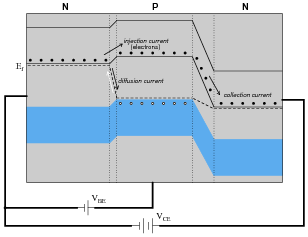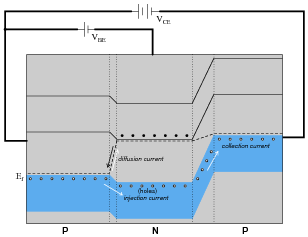An analogy to help illustrate this is a person tossing flower petals into the air above their head, while a breeze carries the petals horizontally away from them. None of the flower petals may be ßwept” away by the breeze until the person releases them into the air, and the velocity of the breeze has no bearing on how many flower petals are swept away from the person, since they must be released from the person’s grip before they can go anywhere.

By referencing either the energy diagram or the flower petal analogy, explain why the collector current for a BJT is strongly influenced by the base current and only weakly influenced by the collector-to-emitter voltage.

• #### Question 7

Bipolar junction transistor (BJT) function is usually considered in terms of currents: a relatively small current through one of the transistor’s terminals exerts control over a much larger current. Draw the directions of all currents for these two transistors (one NPN and one PNP), clearly identifying which of the currents is doing the control, and which of the currents is being controlled:• #### Question 8

The “beta” ratio (β) of a bipolar junction transistor, sometimes alternatively referred to as hFE, is a very important device parameter. In essence, it describes the amplifying power of the transistor. Give a mathematical definition for this parameter, and provide some typical values from transistor datasheets.

• #### Question 9

Find one or two real bipolar junction transistors and bring them with you to class for discussion. Identify as much information as you can about your transistors prior to discussion:

Terminal identification (which terminal is base, emitter, collector)
Continuous power rating
Typical β
• #### Question 10

Match the following bipolar transistor illustrations to their respective schematic symbols:• #### Question 11

If we were to compare the energy diagrams for three pieces of semiconducting material, two “N” type and one “P” type, side-by-side, we would see something like this: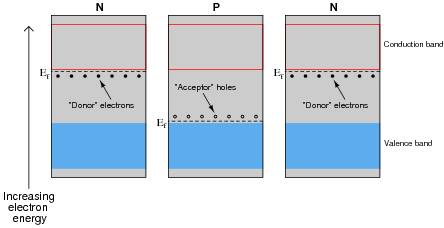The presence of dopants in the semiconducting materials creates differences in the Fermi energy level (Ef) within each piece.

Draw a new energy diagram showing the equilibrium state of the three pieces after being joined together.

• #### Question 12

Transistor operation may be explained in terms of three different currents: injection, diffusion, and collection. Describe what each of these currents are, and how they help explain the amplifying nature of a transistor.

• #### Question 13

Trace the paths of injection, diffusion, and collection currents in this energy diagram for an NPN transistor as it is conducting: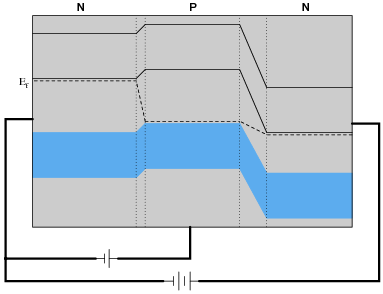• #### Question 14

Trace the paths of injection, diffusion, and collection currents in this energy diagram for a PNP transistor as it is conducting: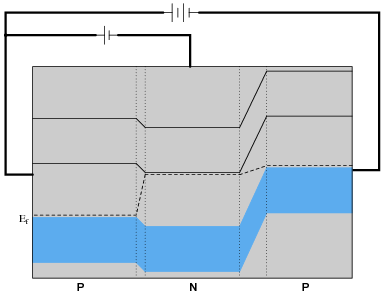• #### Question 15

From an examination of the energy diagram for a BJT in its conducting mode (current existing through each of the three terminals: emitter, base, and collector), determine the biasing of the two PN junctions:

The emitter-base junction (forward or reverse biased?)
The base-collector junction (forward or reverse biased?)

One of these two junctions actually operates in the reverse-bias mode while the transistor is conducting. Explain how this is possible, as a simple PN junction (a diode) operating in reverse-bias mode conducts negligible current.

• #### Question 16

Bipolar junction transistors are classified as minority carrier devices. Explain why.

• #### Question 17

Transistors act as controlled current sources. That is, with a fixed control signal in, they tend to regulate the amount of current going through them. Design an experimental circuit to prove this tendency of transistors. In other words, how could you demonstrate this current-regulating behavior to be a fact?

• #### Question 18

Compare the relative magnitudes of each current in this bipolar transistor circuit:Which current is the smallest and which is the largest? Are there any two currents that are closer in magnitude than with the third? If so, which currents are they?

• #### Question 19

Are the collector and emitter terminals of a transistor interchangeable? If not, what is the physical difference between the emitter and collector?

• #### Question 20

A beginning electronics student is just learning about transistors, and reads in the textbook that a bipolar transistor (either NPN or PNP) can be thought of as two diodes connected back-to-back as such:Acting on this idea, the student proceeds to connect two 1N4001 rectifying diodes back-to-back and try to use it as a transistor. This idea does not work: although the diode pair reads the same patterns of continuity as a transistor would, it does not amplify. Explain why.

Note: this is a fairly deep question, and may not be answered without an understanding of charge carrier energy levels and semiconductor junction behavior.

• #### Question 21

How would you explain the necessary conditions for conduction of an electric current through a BJT? Describe must be done to a BJT in order for it to conduct a current.

• #### Question 22

Draw the polarities ( and -) of the applied voltages necessary to turn both these transistors on:Also, draw the direction of the controlled current (flowing between collector and emitter) that will result from a power source properly connected between these terminals.

• #### Question 23

Students new to the study of transistors often have difficulty remembering the proper directions of currents through bipolar junction transistors, since there are three different currents (IB, IC, IE) and they must “mesh” through the transistor in a particular way.

Draw the proper current directions for each of these transistors, and explain how you are able to remember the correct directions they go:• #### Question 24

Predict how all three transistor currents (IB, IC, and IE) will be affected as a result of the following faults. Consider each fault independently (i.e. one at a time, no multiple faults):Base resistor RB fails open:
Collector resistor RC fails open:
Solder bridge (short) past base resistor RB:
Solder bridge (short) past collector resistor RC:

For each of these conditions, explain why the resulting effects will occur.

• #### Question 25

Based on these DC continuity tester indications, what type of transistor is this, PNP or NPN?

Resistance with negative test lead on pin 1, positive test lead on pin 2: no continuity
Resistance with negative test lead on pin 1, positive test lead on pin 3: no continuity
Resistance with negative test lead on pin 2, positive test lead on pin 1: no continuity
Resistance with negative test lead on pin 2, positive test lead on pin 3: no continuity
Resistance with negative test lead on pin 3, positive test lead on pin 1: continuity
Resistance with negative test lead on pin 3, positive test lead on pin 2: continuity

Also, to the best of your ability, identify the transistor’s three terminals (emitter, base, and collector).

• #### Question 26

Many digital multimeters have a “diode check” range that allows the user to measure the forward voltage drop of a PN junction: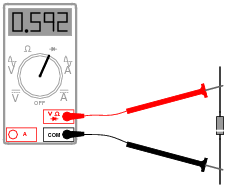When using a multimeter with this feature to identify the terminals of a bipolar junction transistor, the forward voltage drop indication is necessary to distinguish the collector terminal from the emitter terminal. Explain how this distinction is made on the basis of the forward voltage measurement, and also explain why this is.

• #### Question 27

Identify the terminals on this BJT, and also the type of BJT it is (NPN or PNP):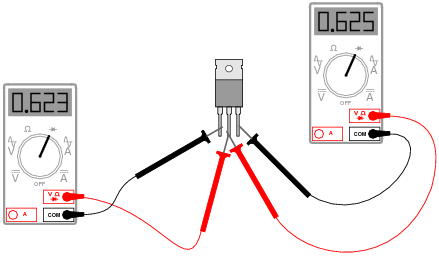• #### Question 28

One of the most important parameters for semiconductor components is the power rating. Explain why power rating is such a critical parameter, especially compared with other types of electronic components (resistors, inductors, capacitors, etc.).•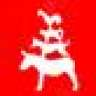LvW November 15, 2019